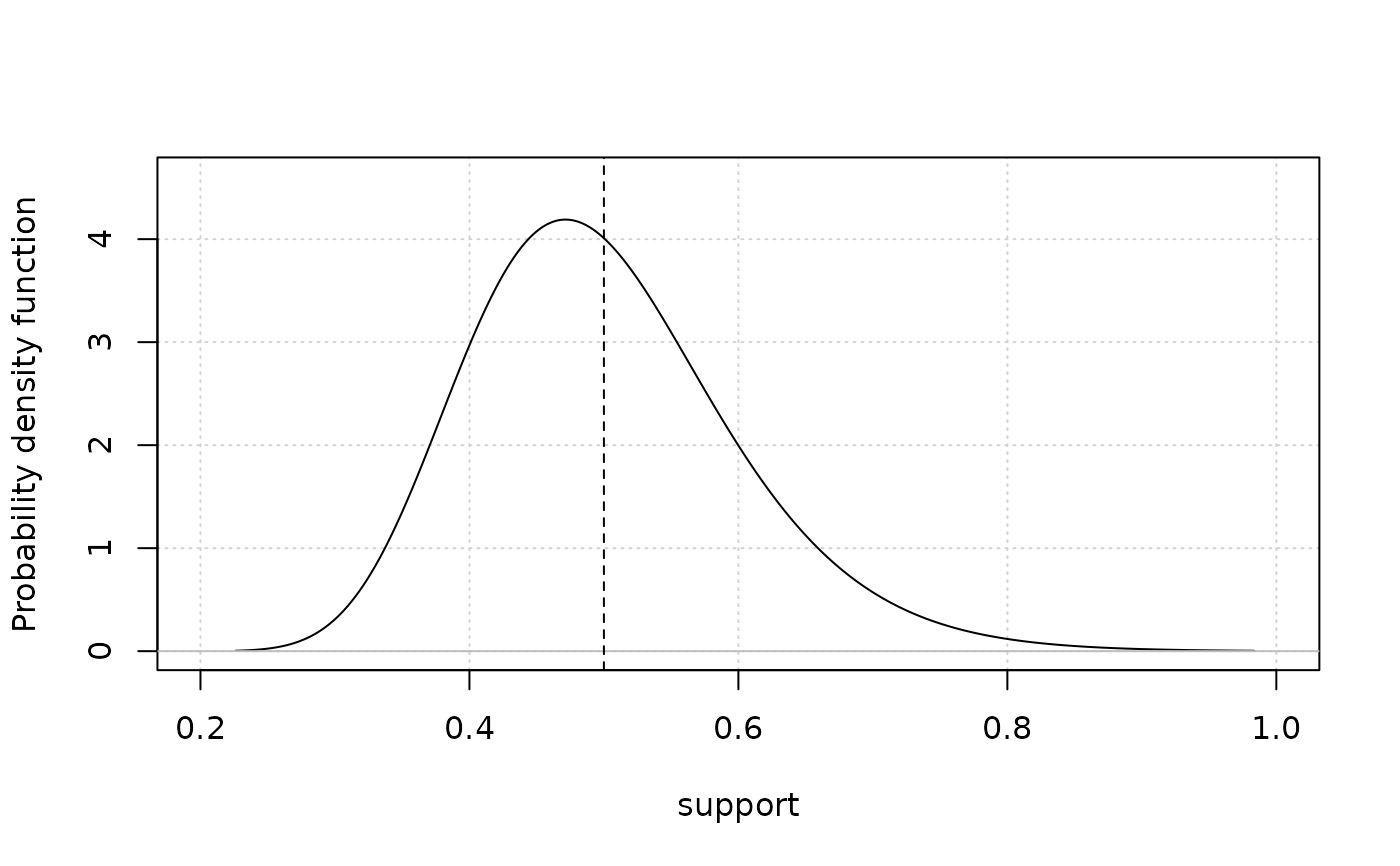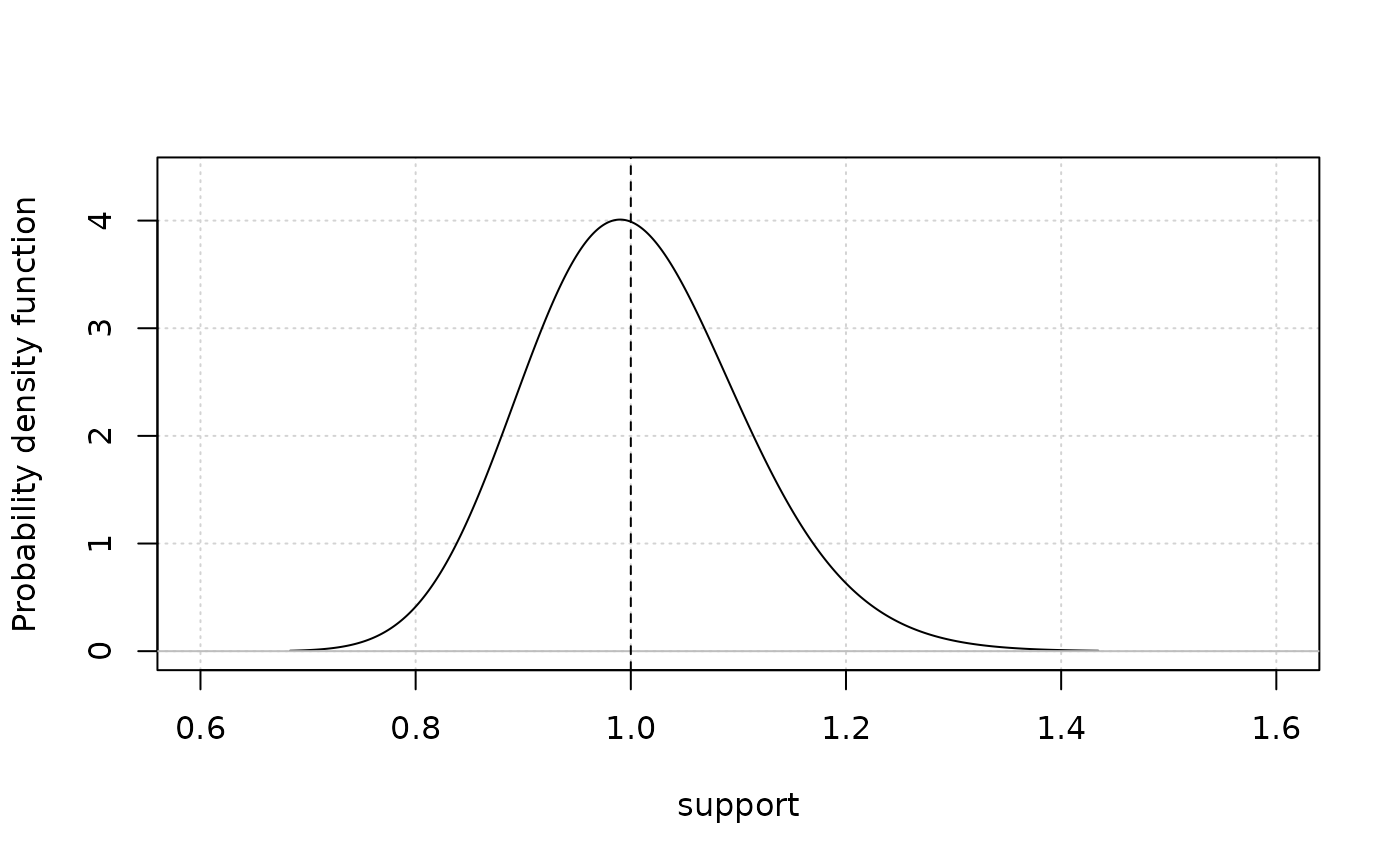Plots the probability distribution function of a lognormal variable from the mean and standard deviation in either transformed (normal) or untransformed space.

plot_lognormalvar(m, sd, label = NULL, logtransform = FALSE, color = "black")

## Arguments

m

A vector of means of the distribution.

sd

A vector of standard deviations of the distribution.

label

Name of the variable to be used as x-axis label.

logtransform

Indicates whether the mean and standard deviation are in lognormal (TRUE) or normal (FALSE) space.

color

A vector of colors.

## Value

A plot of the probability distribution function. Vertical dotted line indicates mean of distribution. This function can plot multiple curves when multiple means and standard deviations are provided.

plot_betavar plot_steepness

Q. Huynh

## Examples

mu <- 0.5
stddev <- 0.1
plot_lognormalvar(mu, stddev) # mean of plot should be 0.5#logtransformed parameters
mu <- 0
stddev <- 0.1
plot_lognormalvar(mu, stddev, logtransform = TRUE) # mean of plot should be 1